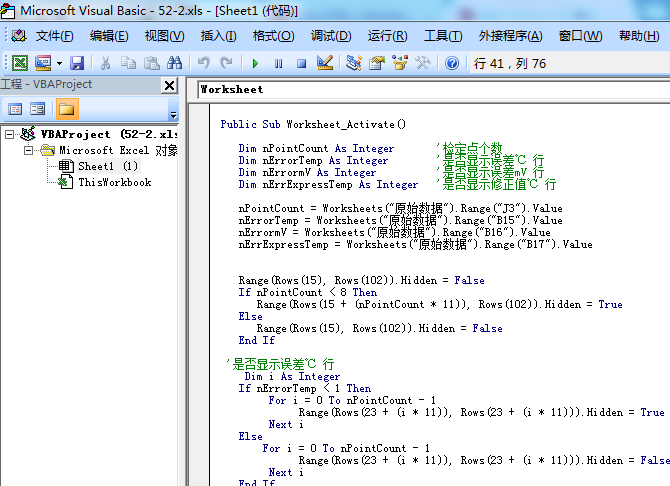# 将多个EXCEL工作簿合并为同一个工作簿的多个不用工作表代码

...全文
418 点赞 收藏 5

5 条回复
ffon123 01月08日

Sub CollectWorkBookDatas()
Dim shtActive As Worksheet, rng As Range, shtData As Worksheet
Dim nTitleRow As Long, k As Long, nLastRow As Long
Dim i As Long, j As Long, nStartRow As Long
Dim aData, aResult, nStarRng As Long
Dim strPath As String, strFileName As String
Dim strKey As String, nShtCount As Long
With Application.FileDialog(msoFileDialogFolderPicker)
'取得用户选择的文件夹路径
If .Show Then strPath = .SelectedItems(1) Else Exit Sub
End With
If Right(strPath, 1) <> "\" Then strPath = strPath & "\"
strKey = InputBox("请输入需要合并的工作表所包含的关键词：" & vbCrLf & "如未填写关键词，则默认汇总全部表格数据", "提醒")
If StrPtr(strKey) = 0 Then Exit Sub '如果点击了取消或者关闭按钮，则退出程序
nTitleRow = Val(InputBox("请输入标题的行数，默认标题行数为1", "提醒", 1))
If nTitleRow < 0 Then MsgBox "标题行数不能为负数。", 64, "警告": Exit Sub
Set shtActive = ActiveSheet
With Application
.ScreenUpdating = False
.DisplayAlerts = False
.AskToUpdateLinks = False
End With
ReDim aResult(1 To 80000, 1 To 1) '声明结果数组
Cells.ClearContents '清空当前表格数据
Cells.NumberFormat = "@" '设置单元格为文本格式
strFileName = Dir(strPath & "*.xls*") '使用Dir函数遍历excel文件
Do While strFileName <> ""
If strFileName <> ThisWorkbook.Name Then '避免同名文件重复打开出错
With GetObject(strPath & strFileName)
'以只读'形式读取文件时，使用getobject会比workbooks.open稍快
For Each shtData In .Worksheets '遍历表
If InStr(1, shtData.Name, strKey, vbTextCompare) Then
'如果表中包含关键字则进行汇总(不区分关键词字母大小写）
Set rng = shtData.UsedRange
If rng.Count > 1 Then '判断工作表是否存在数据……
nShtCount = nShtCount + 1 '汇总工作表的数量
nStartRow = IIf(nShtCount = 1, 1, nTitleRow + 1) '判断遍历数据源是否应该扣掉标题行
aData = rng.Value '数据区域读入数组arr
If UBound(aData, 2) + 2 > UBound(aResult, 2) Then '动态调整结果数组brr的最大列数
ReDim Preserve aResult(1 To UBound(aResult), 1 To UBound(aData, 2) + 2)
End If
For i = nStartRow To UBound(aData) '遍历行
k = k + 1
aResult(k, 1) = strFileName '数组第一列放工作簿名称
aResult(k, 2) = shtData.Name '数组第二列放工作表名称
For j = 1 To UBound(aData, 2) '遍历列
aResult(k, j + 2) = aData(i, j)
Next
If k > UBound(aResult) - 1 Then
'如果数据行数到达结果数组的上限，则将数据导入汇总表，并清空结果数组
With shtActive
nLastRow = .Cells(Rows.Count, 1).End(xlUp).Row '获取放置来源数据的位置
If nLastRow = 1 Then '判断是否扣除标题行
nStarRng = IIf(nTitleRow = 0, 1, 0)
.Range("a1").Offset(nStarRng).Resize(k, UBound(aResult, 2)) = aResult
.Range("a1:b1") = Array("来源工作簿名称", "来源工作表名称")
'前两列放来源工作簿和工作表名称
Else
.Range("a1").Offset(nLastRow).Resize(k, UBound(aResult, 2)) = aResult
'放结果数组的数据
End If
End With
k = 0
ReDim aResult(1 To UBound(aResult), 1 To UBound(aResult, 2))
'重新设置结果数组
End If
Next
End If
End If
Next
.Close False '关闭工作簿
End With
End If
strFileName = Dir '下一个excel文件
Loop
If k > 0 Then
shtActive.Select '激活汇总表
nLastRow = Cells(Rows.Count, 1).End(xlUp).Row '放置数据的位置
If nLastRow = 1 Then '如果汇总表数据为空，说明需要汇总的数据没有超过结果数组的上限
nStarRng = IIf(nTitleRow = 0, 1, 0)
Range("a1").Offset(nStarRng).Resize(k, UBound(aResult, 2)) = aResult
Range("a1:b1") = Array("来源工作簿名称", "来源工作表名称")
Else
Range("a1").Offset(nLastRow).Resize(k, UBound(aResult, 2)) = aResult
End If
End If
With Application
.ScreenUpdating = True
.DisplayAlerts = True
.AskToUpdateLinks = True
End With
MsgBox "一共汇总完成。" & nShtCount & "个工作表", , "孙兴华"
End Sub

hzg303 2019年10月04日

nangongxiaobai 2019年08月05日

VB业余爱好者 2019年08月01日VB业余爱好者 2019年08月01日

4459

1.7w+